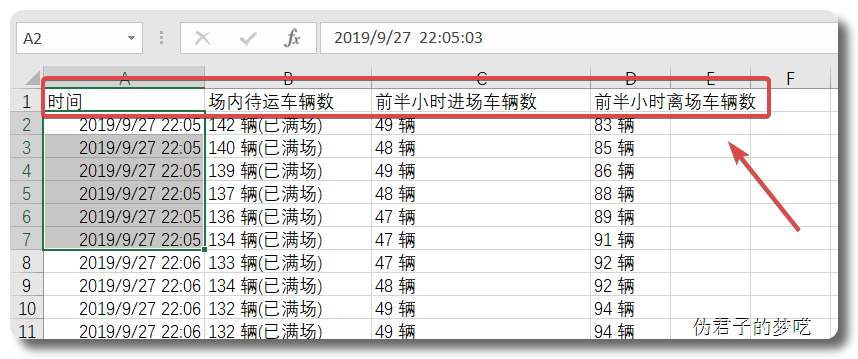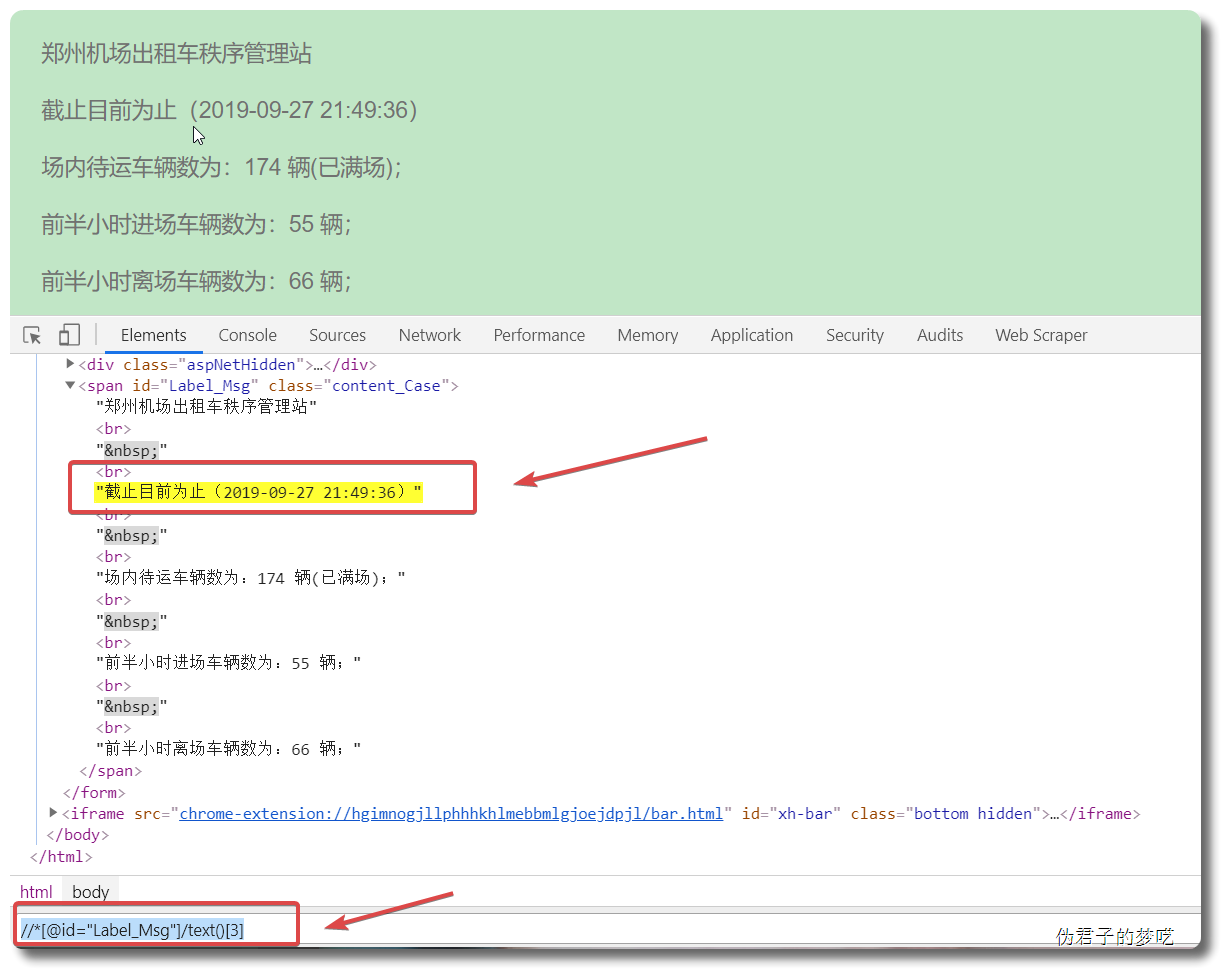## 1 环境说明

Win10 系统下 Python3，编译器是 Pycharm。

## 2 相关代码

import csv
import time
import requests
from lxml import etreewith open("Airport_Taxi-10s.csv", "w", encoding='utf-8-sig', newline="") as f:
csv_write = csv.writer(f)
csv_head = ["时间", "场内待运车辆数", "前半小时进场车辆数", "前半小时离场车辆数"]now_time = now_time.replace("截止目前为止（", "").replace("）", "")

def get_info():
try:
res = requests.get("http://www.whalebj.com/xzjc/default.aspx")
htmlparser = etree.HTML(res.text)

now_time = htmlparser.xpath('//*[@id="Label_Msg"]/text()')
car = htmlparser.xpath('//*[@id="Label_Msg"]/text()')
in_car = htmlparser.xpath('//*[@id="Label_Msg"]/text()')
out_car = htmlparser.xpath('//*[@id="Label_Msg"]/text()')

now_time = now_time.replace("截止目前为止（", "").replace("）", "")
car = car.replace("场内待运车辆数为：", "").replace("；", "")
in_car = in_car.replace("前半小时进场车辆数为：", "").replace("；", "")
out_car = out_car.replace("前半小时离场车辆数为：", "").replace("；", "")

print(now_time, car, in_car, out_car)

row = [now_time, car, in_car, out_car]
out = open("Airport_Taxi-10s.csv", "a+", encoding='utf-8-sig', newline="")
csv_writer = csv.writer(out, dialect="excel")
csv_writer.writerow(row)

out.close()
print()

except Exception as e:
print(e)


while True:
get_info()
time.sleep(10)